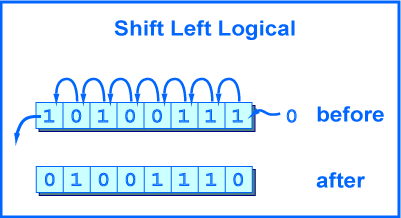# Java and “& 0xFF” exampleBefore you understand what is `& 0xFF`, make sure you know following stuffs :

1. Bitwise AND operator, link.
2. Converts hex to/from binary, and decimal to/from binary.

In short, `& 0xFF` is used to make sure you always get the last 8 bits. Let’s see an example to convert an IP address to/from decimal number.

## 1. Convert IP Address To Decimal

It’s a common practice to convert IpAddress to decimal and store it into database for better calculation and comparison.

``````
Testing IP address = 192.168.1.2
Decimal Number     = 3232235778
``````

To convert `192.168.1.2` to decimal (base 10) the formula is:

``````
192 x (256)^3 + 168 x (256)^2 + 1 x (256)^1 + 2 (256)^0 = ?
3221225472 + 11010048 + 256 + 2 = 3232235778
``````

P.S A standard IP is “base 256”, source.

## 2. Convert Decimal to IP Address, with & 0xFF

To convert decimal back to an IP address, we use bit shifting operator and “mask” it with `& 0xff`.

Java code
``````
long ipAddress = 3232235778L;
String binary = Long.toBinaryString(ipAddress);
System.out.println(binary);
``````

Output

``````
11000000101010000000000100000010
192
168
1
2
``````

The question is why `(ipAddress>>24) & 0xFF` will return 192? Let dive into the binary bit shifting theory below :

2.1 (ipAddress>>24) & 0xFF = 192

``````
Decimal   = 3232235778
Binary    = 11000000 10101000 00000001 00000010
IpAddress = 192      168      1        2

-------------------------->24
Binary    = 00000000 00000000 00000000 11000000

Binary    = 00000000 00000000 00000000 11000000
& 0xFF    = 00000000 00000000 00000000 11111111
Result    = 00000000 00000000 00000000 11000000 = 192 (2^7 + 2^6)
``````

In this case, the 0xFF is optional.

2.2 (ipAddress>>16) & 0xFF = 168

``````
Decimal = 3232235778
Binary  = 11000000 10101000 00000001 00000010

----------------->16
Binary  = 00000000 00000000 11000000 10101000

Binary  = 00000000 00000000 11000000 10101000 = 49320 (2^14 + 2^15 + 2^7 + 2^5 + 2^3)
& 0xFF  = 00000000 00000000 00000000 11111111
Result  = 00000000 00000000 00000000 10101000 = 168
``````

Before `& 0xFF`, you get 49320, after `& 0xFF`, you get the correct 168. Now, you should understand the usage of `& 0xFF`, it just makes sure you always get the last 8 bits.

2.3 (ipAddress>>8) & 0xFF = 1

``````
Decimal = 3232235778
Binary  = 11000000 10101000 00000001 00000010

-------->8
Binary  = 00000000 11000000 10101000 00000001

Binary  = 00000000 11000000 10101000 00000001 = 12625921
& 0xFF  = 00000000 00000000 00000000 11111111
Result  = 00000000 00000000 00000000 00000001 = 1
``````

2.4 (ipAddress) & 0xFF = 2

``````
Decimal = 3232235778
Binary  = 11000000 10101000 00000001 00000010

Binary  = 11000000 10101000 00000001 00000010

Binary  = 11000000 10101000 00000001 00000010 = 3232235778
& 0xFF  = 00000000 00000000 00000000 11111111
Result  = 00000000 00000000 00000000 00000010 = 2
``````

## 3. Java Source Code

Full Java example to demonstrate above scenario.

BitwiseExample.java
``````
package com.mkyong.core;

public class BitwiseExample {

public static void main(String[] args) {

BitwiseExample obj = new BitwiseExample();
long ipAddressInLong = obj.ipToLong("192.168.1.2");

String binary = Long.toBinaryString(ipAddressInLong);
printPrettyBinary(binary);

}

public long ipToLong(String ipAddress) {

long num = 0;
for (int i = 0; i < addrArray.length; i++) {

int power = 3 - i;

// 1. (192 % 256) * 256 pow 3
// 2. (168 % 256) * 256 pow 2
// 3. (2 % 256) * 256 pow 1
// 4. (1 % 256) * 256 pow 0
num += ((Integer.parseInt(addrArray[i]) % 256 * Math.pow(256, power)));

}

return num;
}

public String longToIp(long i) {

return ((i >> 24) & 0xFF) + "." + ((i >> 16) & 0xFF) + "." + ((i >> 8) & 0xFF) + "." + (i & 0xFF);

}

//print pretty binary code, padding left zero
private static void printPrettyBinary(String binary) {

String s1 = String.format("%32s", binary).replace(' ', '0');
System.out.format("%8s %8s %8s %8s %n",
s1.substring(0, 8), s1.substring(8, 16),
s1.substring(16, 24), s1.substring(24, 32));
}

}
``````

Output

``````
3232235778
11000000 10101000 00000001 00000010
192.168.1.2
``````

Photo Credit : http://chortle.ccsu.edu/AssemblyTutorial/Chapter-12/lshiftLeft.gif

## References

#### About the Author##### mkyong
Founder of Mkyong.com, love Java and open source stuff. Follow him on Twitter. If you like my tutorials, consider make a donation to these charities.0 Followers

Most reacted comment
8 Comment authorsRecent comment authorsGuest
MrfksIv

This was very helpful while trying to understand how bufferedImages in Java work! Thanks a lotGuest
niranga sandaruwan

really helpful article.one of the best programming tutorial site i have seen so far.keep up your works.Guest
Rasmita Patra

nice article with clear explanation…..Guest
Apoorva

Thank you, it was very helpfulGuest
Nik

Perfect. Thanks :)Guest
uNiverselEgacy

Should mention that & 0xFF is also helpful to prevent sign extension.
int i = (byte)0xAB;// i == -85
int i = (byte)0xAB & 0xFF; // i == 171Guest
Suraj

The reason why binaryIP>>24=192 is because when we convert IP address to decimal we are actually doing

``` 192 * (256)^3 = 192 * (2^8)^3= 192 * (2^24) ```

So, we are, basically, left shifting by 24 places here. Hence, right shifting 24 places gives the original resultGuest
Ecka

thank you for a good and educational article